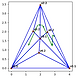top of page

# Game Theory Notebooks

## Zero-sum games

• Matrix games

• Minimax problems and saddle points

• LP formulation## Scarf's algorithm

• Simplical subdivisions

• Labelled simplices

• Path-following algorithm## Linear complementarity problems (LCPs)

• Basic theory of LCPs

• Computation using Lemke’s algorithm

• Computation using gurobi## Computation of Nash equilibria

• Nash equilibrium in a bimatrix games as a LCP

• Mangasarian-Stone computation of Nash equilibria## Accessing the material

Github repository

bottom of page